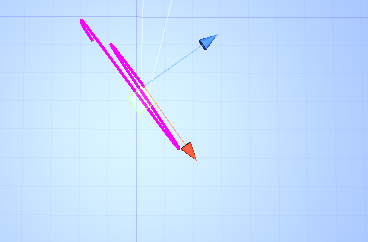# Why is my character controller moving in unrelated directions?

I tested my movement script with the following code (condensed for length), and without ever pressing “W” or “S,” the player somehow manages to move forwards. I have also attached two images. In both images, the character controller’s path is portrayed by a line renderer which follows the player. Additionally, in both cases the player only presses “A” and “D.” In the second image, the player is rotated by exactly 54.609 degrees on the y axis. The difference being that when facing completely forwards, the issue where the player moves forward does not occur; why is the player moving forwards when pressing a/d as depicted in image 2?
_
I am attempting to avoid using transformDirection(velocity) on every characterController.Move() call, so that the player continues travelling in the same direction regardless of the rotation of the player, which is more or less the law of inertia.``````if (Input.GetKey(KeyCode.W))
{
velocity += xzMover.transform.forward * accelerationSpeed * Time.deltaTime;
}
if (Input.GetKey(KeyCode.S))
{
velocity -= xzMover.transform.forward * accelerationSpeed * Time.deltaTime;
}
if (Input.GetKey(KeyCode.D))
{
velocity += xzMover.transform.right * accelerationSpeed * Time.deltaTime;
}
if (Input.GetKey(KeyCode.A))
{
velocity -= xzMover.transform.right * accelerationSpeed * Time.deltaTime;
}
playerController.move(velocity * Time.deltaTime);
``````

Edit: Tested it with TransformDirection(x, 0, z), and got exactly the same results. For each keypresses, the velocity increased by transformDirection(accelerationSpeed, 0, accelerationSpeed), replacing either the x or the z with 0 depending on whether the player was increasing velocity on the x or z axis.

I would simply assume that you move on a slope? So you naturally move down the slope due to the collision with the ground.